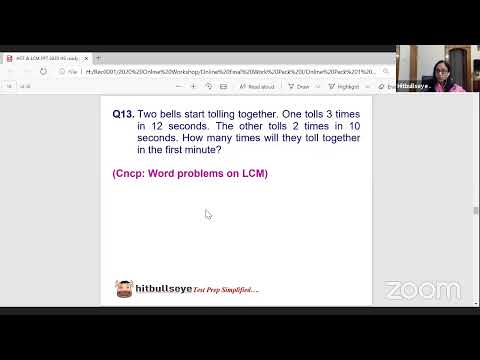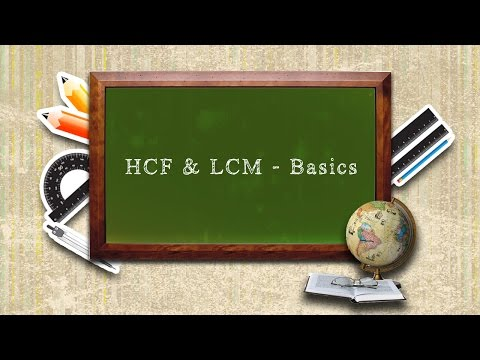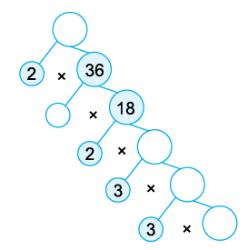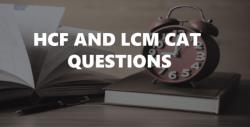Courses

HCF And LCM - MCQ 1

10 Questions MCQ Test Quantitative Techniques for CLAT | HCF And LCM - MCQ 1

Description
Attempt HCF And LCM - MCQ 1 | 10 questions in 20 minutes | Mock test for Quant preparation | Free important questions MCQ to study Quantitative Techniques for CLAT for Quant Exam | Download free PDF with solutions
QUESTION: 1

Six bells commence tolling together and toll at intervals of 2, 4, 6, 8, 10 and 12 seconds respectively. In 30 minutes, how many times do they toll together?

Solution:

L.C.M. of 2, 4, 6, 8, 10, 12 is 120.So, the bells will toll together after every 120 seconds, i.e, 2 minutes.In 30 minutes, they will toll together 30/2 + 1 = 16

QUESTION: 2

The least multiple of 7, which leaves a remainder of 4, when divided by 6, 9, 15 and 18 is:

Solution:

L.C.M. of 6, 9, 15 and 18 is 90.
Let required number be 90k + 4, which is multiple of 7.
Least value of k for which (90k + 4) is divisible by 7 is k = 4.
Required number = (90 x 4) + 4 = 364.

QUESTION: 3

The least number, which when divided by 48, 60, 72, 108 and 140 leaves 38, 50, 62, 98 and 130 as remainders respectively, is:

Solution:

Here (48 – 38) = 10, (60 – 50) = 10, (72 – 62) = 10, (108 – 98) = 10 & (140 – 130) = 10.
Required number = (L.C.M. of 48, 60, 72, 108, 140) – 10
= 15120 – 10 = 15110

QUESTION: 4

The H.C.F. of two numbers is 11 and their L.C.M. is 7700. If one of the numbers is 275, then the other is:

Solution:

Other number =[11 x 7700]/275 = 308

QUESTION: 5

A, B and C start at the same time in the same direction to run around a circular stadium. A completes a round in 252 seconds, B in 308 seconds and C in 198 seconds, all starting at the same point. After what time will they meet again at the starting point?

Solution:

L.C.M. of 252, 308 and 198 = 2772.So, A, B and C will again meet at the starting point in 2772 see i.e., 46 min. 12 sec

QUESTION: 6

The L.C.M. of two numbers is 48. The numbers are in the ratio 2 : 3. Then sum of the number is:

Solution:

Let the numbers be 2x and 3x.
Then, their L.C.M. = 6x.
So, 6x = 48 or x = 8.
The numbers are 16 and 24.
Hence, required sum = (16 + 24) = 40.

QUESTION: 7

The least number, which when divided by 12, 15, 20 and 54 leaves in each case a remainder of 8 is:

Solution:

Required number = (L.C.M. of 12, 15, 20, 54) + 8
= 540 + 8
= 548.

QUESTION: 8

The product of two numbers is 4107. If the H.C.F. of these numbers is 37, then the greater number is:

Solution:

Let the numbers be 37a and 37b. Then, 37a x 37b = 4107
ab = 3.
Now, co-primes with product 3 are (1, 3).
So, the required numbers are (37 x 1, 37 x 3) i.e., (37, 111).
Greater number = 111.

QUESTION: 9

The product of two numbers is 2028 and their H.C.F. is 13. The number of such pairs is:

Solution:

Let the numbers 13a and 13b.
Then, 13a x 13b = 2028
⇒ ab = 12.
Now, the co-primes with product 12 are (1, 12) and (3, 4).
[Note: Two integers a and b are said to be coprime or relatively prime if they have no common positive factor other than 1 or, equivalently, if their greatest common divisor is 1 ]
So, the required numbers are (13 x 1, 13 x 12) and (13 x 3, 13 x 4).
Clearly, there are 2 such pairs.

QUESTION: 10

The least number which should be added to 2497 so that the sum is exactly divisible by 5, 6, 4 and 3 is:

Solution:

L.C.M. of 5, 6, 4 and 3 = 60. On dividing 2497 by 60, the remainder is 37. Number to be added = (60 – 37) = 23Use Code STAYHOME200 and get INR 200 additional OFF Use Coupon Code

Track your progress, build streaks, highlight & save important lessons and more!

Similar ContentRelated tests